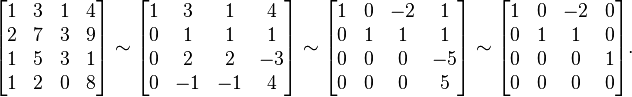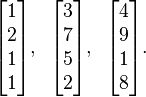Basis

The columns of A span the column space, but they may not form a basis if the column vectors are not linearly independent. Fortunately, elementary row operations do not affect the dependence relations between the column vectors. This makes it possible to use row reduction to find a basis for the column space.

For example, consider the matrixThe columns of this matrix span the column space, but they may not be linearly independent, in which case some subset of them will form a basis. To find this basis, we reduce A to reduced row echelon form:At this point, it is clear that the first, second, and fourth columns are linearly independent, while the third column is a linear combination of the first two. (Specifically, v3 = –2v1 + v2.) Therefore, the first, second, and fourth columns of the original matrix are a basis for the column space:Note that the independent columns of the reduced row echelon form are precisely the columns with pivots. This makes it possible to determine which columns are linearly independent by reducing only to echelon form.

The above algorithm can be used in general to find the dependence relations between any set of vectors, and to pick out a basis from any spanning set. A different algorithm for finding a basis from a spanning set is given in the row space article; finding a basis for the column space of A is equivalent to finding a basis for the row space of the transpose matrix AT.1.Belajar Komputer